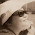## Wednesday, 13 February 2013

### What's Wrong?

As discussed in class, something is not quite right on the working.
Are you able to tell what's wrong?

1.The bracket was wrongly placed in the working area. If you follow the working, it will give you the wrong answer by using 2 brackets for 2/3 and 1/5. The subtraction of 2/3 and 1/5 should be squared together and not separately.

1.You have correctly pointed out that we should follow the "law" of operations :)
Should work with the terms within the brackets first.

2.The answer given was wrong as the subtraction should be done before squaring them.
We should not be using this method as there is a formula for the above equation.
The formula as follows:

(a - b)^2 = a^2 - 2ab + b^2
(2/3 - 1/5)^2 = (2/3)^2 - 2(2/3)(1/5) + (1/5)^2
= 4/9 - 4/15 + 1/25
= 100/225 - 60/225 + 9/225
= 49/225

1.Good explanation that illustrates how such misconceptions can be explained using Algebra :)

What Lavinn has described is one of the Special Products of Algebra (Expansion) that we will be learning in Term 2/3 :)

Subsequently, we'll see how Algebra will help us to understand/ uncover some of the "mysteries" in real life :)

3.1/5^2 is not equal to 1/5. It should be 1/25. This mistake affected the rest of the working. The overall answer should be 49/225

1.You have also pointed out another mistake, that the term (1/5)^2 was correctly evaluated.
On top of that, the first step is already incorrect - we can't just square the terms individually.

4.The subtraction should have been done before squaring the numbers. And like what Pei Ling said, the mistake affected the rest of the working.

1.Yes, you can correctly pointed out the order of operation was not followed in the working :)

5.The subtraction should be done first (Order of operations).

1.Yes, we should work on the terms within the bracket first :)